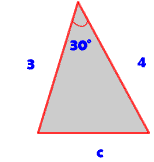# Solve for missing parts of a triangle

### Question:

My teacher wants me to find the sides of a triangle, but it's not a right triangle. I know two sides and one of the angles. How can I find the third side? Can I use the Pythagorean Theorem?$$c^2 = a^2 + b^2 - 2ab\cos{C}$$
$$c^2 = a^2 + b^2 - 2ab\cos{C}$$ $$c^2 = 3^2 + 4^2 - 2*3*4*\cos{30°}$$ $$c^2 = 25 - 24*\frac{\sqrt{3}}{2}$$ $$c = 2.05$$